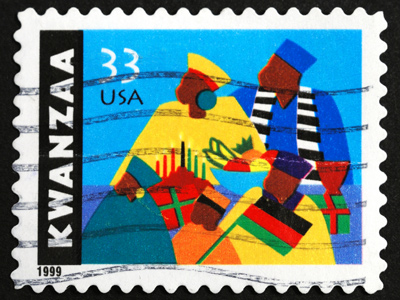33 is the biggest number you can divide into both the numerator and denominator of 33/66.

# Numbers - Fractions - Simplification

This Math quiz is called 'Numbers - Fractions - Simplification' and it has been written by teachers to help you if you are studying the subject at middle school. Playing educational quizzes is a fabulous way to learn if you are in the 6th, 7th or 8th grade - aged 11 to 14.

It costs only \$12.50 per month to play this quiz and over 3,500 others that help you with your school work. You can subscribe on the page at Join Us

Simplification of fractions means looking for the highest common factor on the top and bottom numbers of the fraction in order to write the fraction in its simplest form.

By the time you've finished this Math quiz, you'll be a dab hand at the subject!

1.
What is the simplest way of writing 1/2?
1/4
1/3
1/2
1/5
1/2 is in its simplest form already!
2.
What is the biggest number you can divide into both the numerator and denominator of 33/66?
3
6
11
33
Although both numbers are divisible by 11, it isn't the HIGHEST common factor
3.
How would you write 9/81 in its lowest terms?
1/9
9/1
9/8
It's not possible
Both numbers are divisible by 9
4.
What is 30/40 as a fraction in its simplest form?
1/2
3/4
30/40
300/400
5.
What is the biggest number you can divide into both the numerator and denominator of 30/40?
3
4
10
There isn't one
Dividing 30 (the numerator) and 40 (the denominator) by 10 gives us 3/4
6.
How would you write 33/66 in its simplest form?
33/66
3/6
1/2
3/4
'Simplest form' is also known as 'lowest terms'
7.
How would you write 10/100 in its simplest form?
1/10
1/100
100/10
10/1
The common factor is 10
8.
What is the biggest number you can divide into both the numerator and denominator of 12/18?
3
6
9
12
9.
What is the biggest number you can divide into both the numerator and denominator of 71/92?
1/9
7/9
7/2
It's not possible
There is no common factor!
10.
How would you write 12/18 in its simplest form?
1/3
2/3
3/4
5/10
Author:  Frank Evans